Our prices are denominated in USD.

We ship internationally!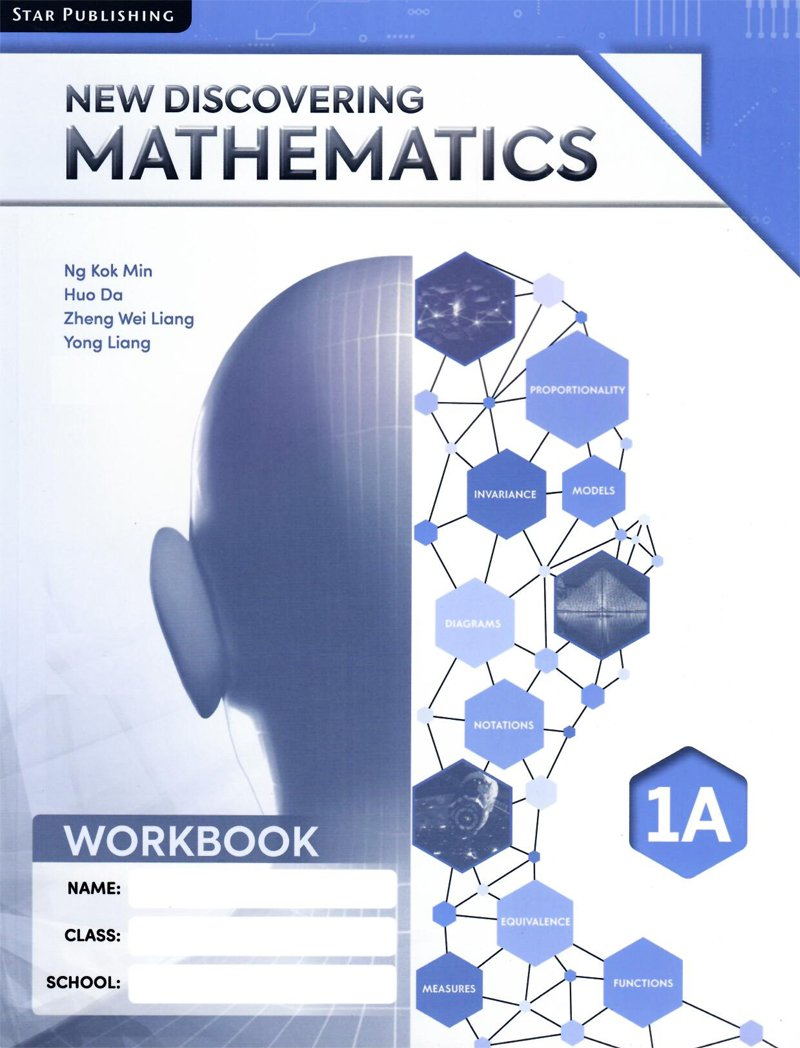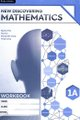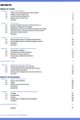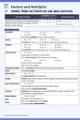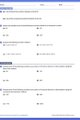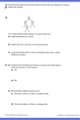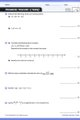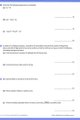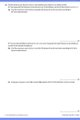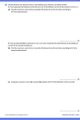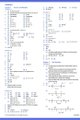# New Discovering Mathematics Workbook 1A

US\$8.65

New Discovering Mathematics is a series of textbooks written with a learner-centric approach that builds upon the knowledge and skills learnt in primary school. It is based on the latest O-level Mathematics syllabus implemented from 2020 by the Ministry of Education of Singapore.

The emphasis of this series is on empowering students to learn mathematics independently and effectively. Depending on the topics covered, different approaches are used to present different concepts to facilitate students' understanding.

New Discovering Mathematics is organised into three content strands: Numbers & Algebra, Geometry & Measurement and Statistics & Probability.

For Secondary 1 / Grade 7 / Year 7 / 13 years old

Singapore Curriculum

1. Factors and Multiples
Primes, Factors, Multiples, Roots

2. Real Numbers
Negative numbers, Number Line, Integers, Operations, Rational and Real Numbers

3. Approximation and Estimation
Rounding off to Decimal Places and Significant Figures, Estimation

4. Basic Algebra & Algebraic Manipulation
Use of Letters in Algebra, Algebraic Expressions and Formulae
Like, Unlike Terms, Simplification and Factorisation of Linear Expressions

Revision Exercise 1

5. Simple Equations in One Variable
Simple Linear Equations in One Variable, Brackets, Simple Fractional Equations, Forming Equations

6. Angles and Parallel Lines
Points, Lines, Planes, Angles, Parallel Lines, Perpendicular and Angle Bisectors

7. Triangles and Polygons